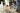# Understanding Ratios and Proportions Plus Proportional Relationships

POSTED ON MARCH 30, 2023Ratios and proportions are essential concepts to learn in math and in real life. Explore the key components of ratios and learn how you can compare different quantities using fractions and ratios.

## What Are Ratios and Proportions?

Ratios are used to compare two (or more) quantities by writing them as a/b or a:b, where a and b are the two quantities being compared. The colon symbol, :, separates the two quantities. Meanwhile, proportions highlight two or more equal ratios, showing the relationship between groups of quantities. Watch this video about ratios and understand what they represent.

Juni Learning has excellent resources such as the video you see in this article. These multimedia resources offer a safe space for you to explore new techniques and methods. Explore Juni Learning’s content to support you in mastering Common Core math topics and concepts. Juni Learning will not only help you in math, but its entire catalog can help you with other subjects too!Take a look at the jars of honey and strawberry jams shown above. As you have learned, you can write quantities in ratio to highlight the relative amount of honey and jams present in each group. There are 3 jars of honey for every 4 jars of jams, so the ratio of honey to jam is 3:4.You can also switch these quantities over if what’s needed is the number of jams to honey instead, the ratio of jam to honey is 4:3. Now, it’s time for you test your understanding with this example.

Our advisors can answer any questions you have about our curriculum or subscriptions. They can even provide a course recommendation.

#### Example 1Jacob enjoys reading books (both fiction and nonfiction). In his home library, he counted the total number of math, science, history, and geography books. The image above represents the books present in Jacob’s library. Write down the ratio of the following quantities:

a. math books to science books

There are 9 math books and 5 science books, so the ratio between math books to science books is 9:5.

b. science books to history books to geography booksApplying a similar process, the ratio between science books to history books to geography books is equal to 5:4:9.

c. math books to total number of books

Yes, it’s possible to write ratios to highlight the portion that a particular quantity makes up the whole. Now, take a look at the number of math books and the total number of books from Jacob’s library.Add up all the number of books then compare this to the number of math books.Lastly, the ratio between science books to history books to geography books is equal to 9:27 (or 1:3). You’re doing great with ratios so far, so why don’t we level this up by understanding what equivalent ratios are and how they are helpful with proportions.

## Understanding Equivalent Ratios

Equivalent ratios are ratios that are identical once they are simplified. Like equivalent fractions, equivalent ratios will simplify to one identical ratio. When comparing quantities, their ratios can be simplified or scaled up depending on the given factor.Take a look at the pink and yellow tiles shown above. As can be seen, there are 12 pink tiles for every 4 yellow tiles. This relationship applies throughout the four rows. In fact, for each row, you’ll see that there are 3 pink tiles for every 1 yellow tile.

This confirms that 12:4 and 3:1 are equivalent ratios. You can also double-check this by dividing 12:4 by 4 for each quantity to simplify it or multiplying 3:1 by 4 to scale it up.Yes, these are like looking for equivalent fractions! Let’s now see how proportions and how ratios can help with proportional relationships.

Boost math confidence to the next level
Juni’s vetted instructors study at top US Universities and provide our students with the support and mentorship to grow their math skills.

## What Is a Proportional Relationship?

A proportional relationship occurs when two or more quantities share equivalent ratios. Equivalent ratios share a common factor and it’s time to put a name on it: the constant of proportionality. Why don’t we begin with simpler examples?Let’s say a recipe for a batch of chocolate brownies requires 2 slabs of butter for every 3 bars of chocolate. Even when the recipe is tweaked to bake more brownies, the ratio will remain the same. For two batches of brownies, you’ll need 2 x 2 = 4 slabs of butter for every 3 x 2 = 6 bars of chocolate.Meaning, the ingredients from recipes like this will share proportional relationships. Suppose that the number of butter slabs and chocolate bars are graphed on a xy-plane as shown below.Quantities that share proportional relationships will reflect a linear relationship on the xy-plane. As expected, the quantities or variables will form a straight line when graphed on the coordinate system.